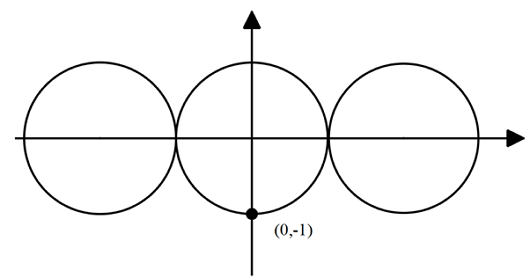# The Farthest Point

Geometry Level 5Consider the three unit circles above with the first and third ones touching the middle one and all their centers on the $x$-axis. From the point $(0,-1)$, locate the point on each of the other circles farthest from it. Connect these three points to form a triangle.

The most simplified ratio of the area of triangle to the sum of areas of the three circles can be expressed in this form

$\dfrac a{b\pi} ( c + \sqrt d) ,$

where $a,b,c$ and $d$ are positive integers with $a,b$ coprime and $d$ square-free. Find $a+b+c+d$.

×